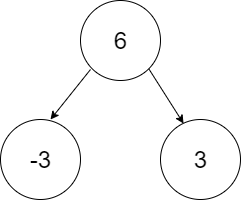New update is available. Click here to update.

# Collect Leaves

Last Updated: 25 Mar, 2021
Difficulty: Moderate

## PROBLEM STATEMENT

#### You are given a binary tree with ‘N’ nodes. Each node has an integer value associated with it. Your task is to print the leaf nodes and then remove these leaf nodes. Now, print the new leaf nodes and repeat the process until the tree becomes empty.

##### Note:
``````You don’t require to remove the leaf nodes. Just print the values in the required order.

The leaf nodes must be printed in a left to right order.
``````
##### Input Format:
``````The first line contains an integer ‘T’, which denotes the number of test cases to be run. Then, the T test cases follow.

The first and only line of each test case contains elements of the binary tree in the level order form. The line consists of values of nodes separated by a single space. In case a node is null, we take -1 in its place.

For example, the input for the tree depicted in the below image would be :
````````````6
-3 3
-1 -1 -1 -1
``````

#### Explanation :

``````Level 1 :
The root node of the tree is 6

Level 2 :
Left child of 6 = -3
Right child of 6 = 3

Level 3 :
Left child of -3 = null (-1)
Right child of -3 = null (-1)
Left child of 3 = null (-1)
Right child of 3 = null (-1)

The first not-null node (of the previous level) is treated as the parent of the first two nodes of the current level. The second not-null node (of the previous level) is treated as the parent node for the next two nodes of the current level and so on.
The input ends when all nodes at the last level are null (-1).
``````
##### Note:
``````The above format was just to provide clarity on how the input is formed for a given tree.
The sequence will be put together in a single line separated by a single space. Hence, for the above-depicted tree, the input will be given as:

6 -3 3  -1 -1 -1 -1
``````
##### Output Format:
``````For each test case, print in a separate line the leaf nodes of the tree. After removing the current leaf nodes, print the new leaf nodes in a separate line. Keep repeating the process until the tree becomes empty.

Output for each test case will be printed in a separate line.
``````
##### Note:
``````You do not need to print anything. It has already been taken care of. Just implement the given function.
``````
##### Constraints:
``````1 <= T <= 100
1 <= N <= 10^5
-10^9 <= node data <= 10^9, (where node data != -1).

Time Limit: 1 sec
``````